Tutorial on Using Excel Spreadsheet to Obtain Bode Plots and Nyquist Plots
1. Preliminaries:
1. Given: G(s), a transfer function in Laplace domain
2. Required: Frequency response plots corresponding to G(s)
1. Bode Plots.
• These consist of two plots. The first plot is a plot of log modulus (in decibels) versus frequency. The second plot is the phase shift (in degrees) versus frequency. Both plots usually have the frequency in logarithmic scale.
• Using the given transfer function G(s),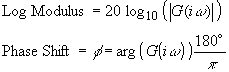1. Nyquist Plots.
• The Nyquist plots is obtained by simply plotting Imaginary(G(iw)) versus Real(G(iw))
For discussion purposes, consider a second order transfer function,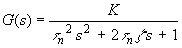1. Set up some cells for the various parameters in the transfer function.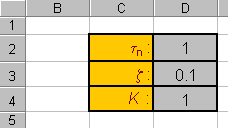1. Next, determine the range of frequencies that are of interest. For example, let 10-1 < w < 101. Since the frequency will be plotted in logarithmic scale, you can use a column to include numbers ranging linearly from -1 to 1, e.g. -1, -0.99,, 0.98, 0.99, 1. Then use another column to evaluate the frequency, e.g. w = 10-1, 10-0.99, , 100.98 100.99, 101.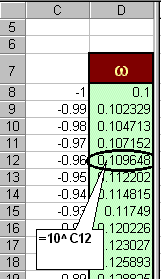1. In the next column, build cells containing complex numbers, s=iw. This can be done by using the

2. COMPLEX( , ) function provided in Excel.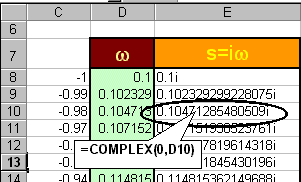(Note: you may need to change the width of the column in order to see the numbers)
1. Now evaluate the transfer function, G(s), with s=iw, using the built-in functions, IMDIV(a,b), IMSUM(a,b), IMPRODUCT(a,b), IMPOWER(a,n) to perform complex division, sum, product and power operations on complex numbers a and b, with n as integer.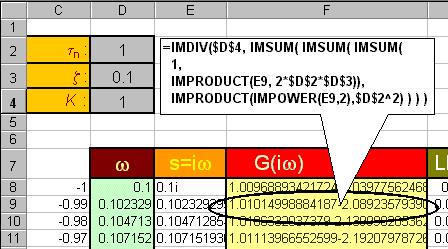1. From the results in G(iw), obtain the Log Modulus and Phase Shift columns: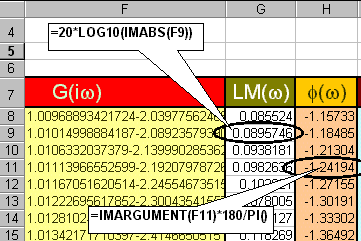1. Also, from G(iw), obtain columns that evaluate Re[G] and Im[G], respectively: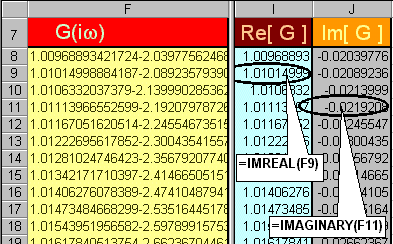1. Using the Log modulus, Phase Shift and Frequency columns, obtains the Bode plots: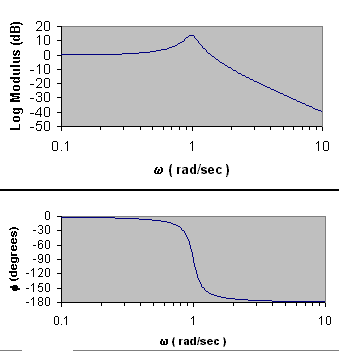1. Using the columns for Re[G] and Im[G], obtain the Nyquist Plot: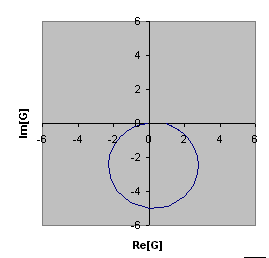This page is maintained by Tomas B. Co (tbco@mtu.edu). Last revised 2/1/00.

Tomas B. Co
Associate Professor
Department of Chemical Engineering
Michigan Technological University
1400 Townsend Avenue
Houghton, MI 49931-1295